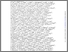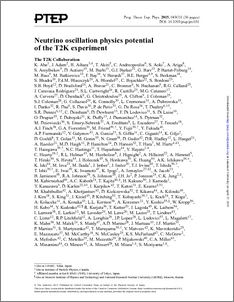# Neutrino oscillation physics potential of the T2K experiment

, T2K Collaboration (2015) Neutrino oscillation physics potential of the T2K experiment. Progress of Theoretical and Experimental Physics, 2015 (4).Preview
PDF (Prog. Theor. Exp. Phys.-2015--)
Prog._Theor._Exp._Phys._2015_.pdf - Published Version

## Abstract

The observation of the recent electron neutrino appearance in a muon neutrino beam and the high-precision measurement of the mixing angle $\theta_{13}$ have led to a re-evaluation of the physics potential of the T2K long-baseline neutrino oscillation experiment. Sensitivities are explored for CP violation in neutrinos, non-maximal $\sin^22\theta_{23}$, the octant of $\theta_{23}$, and the mass hierarchy, in addition to the measurements of $\delta_{CP}$, $\sin^2\theta_{23}$, and $\Delta m^2_{32}$, for various combinations of $\nu$-mode and $$\bar{\nu}$$-mode data-taking. With an exposure of $7.8\times10^{21}$~protons-on-target, T2K can achieve 1-$\sigma$ resolution of 0.050(0.054) on $\sin^2\theta_{23}$ and $0.040(0.045)\times10^{-3}~\rm{eV}^2$ on $\Delta m^2_{32}$ for 100\%(50\%) neutrino beam mode running assuming $\sin^2\theta_{23}=0.5$ and $\Delta m^2_{32} = 2.4\times10^{-3}$ eV$^2$. T2K will have sensitivity to the CP-violating phase $\delta_{\rm{CP}}$ at 90\% C.L. or better over a significant range. For example, if $\sin^22\theta_{23}$ is maximal (i.e $\theta_{23}$=$45^\circ$) the range is $-115^\circ\delta_{\rm{CP}}$+50^\circ\delta_{\rm{CP}}

Item Type:
Journal Article
Journal or Publication Title:
Progress of Theoretical and Experimental Physics
Date of Acceptance: 29/01/2015 © The Author(s) 2015. Published by Oxford University Press on behalf of the Physical Society of Japan. This is an Open Access article distributed under the terms of the Creative Commons Attribution License (http://creativecommons.org/licenses/by/4.0/), which permits unrestricted reuse, distribution, and reproduction in any medium, provided the original work is properly cited. 40 pages, 27 figures, accepted by PTEP
Uncontrolled Keywords:
/dk/atira/pure/subjectarea/asjc/3100
Subjects:
Departments:
ID Code:
73651
Deposited By:
Deposited On:
18 Jun 2015 05:39
Refereed?:
Yes
Published?:
Published